﻿ Probabilistic Programming
val salary : int

Full name: index.salary
val mistake : bool

Full name: index.mistake
val observed : int
Multiple items
val int : value:'T -> int (requires member op_Explicit)

Full name: Microsoft.FSharp.Core.Operators.int

--------------------
type int = int32

Full name: Microsoft.FSharp.Core.int

--------------------
type int<'Measure> = int

Full name: Microsoft.FSharp.Core.int<_>

## How to look like a statistician

### @evelgab# Probabilistic models### Salary distribution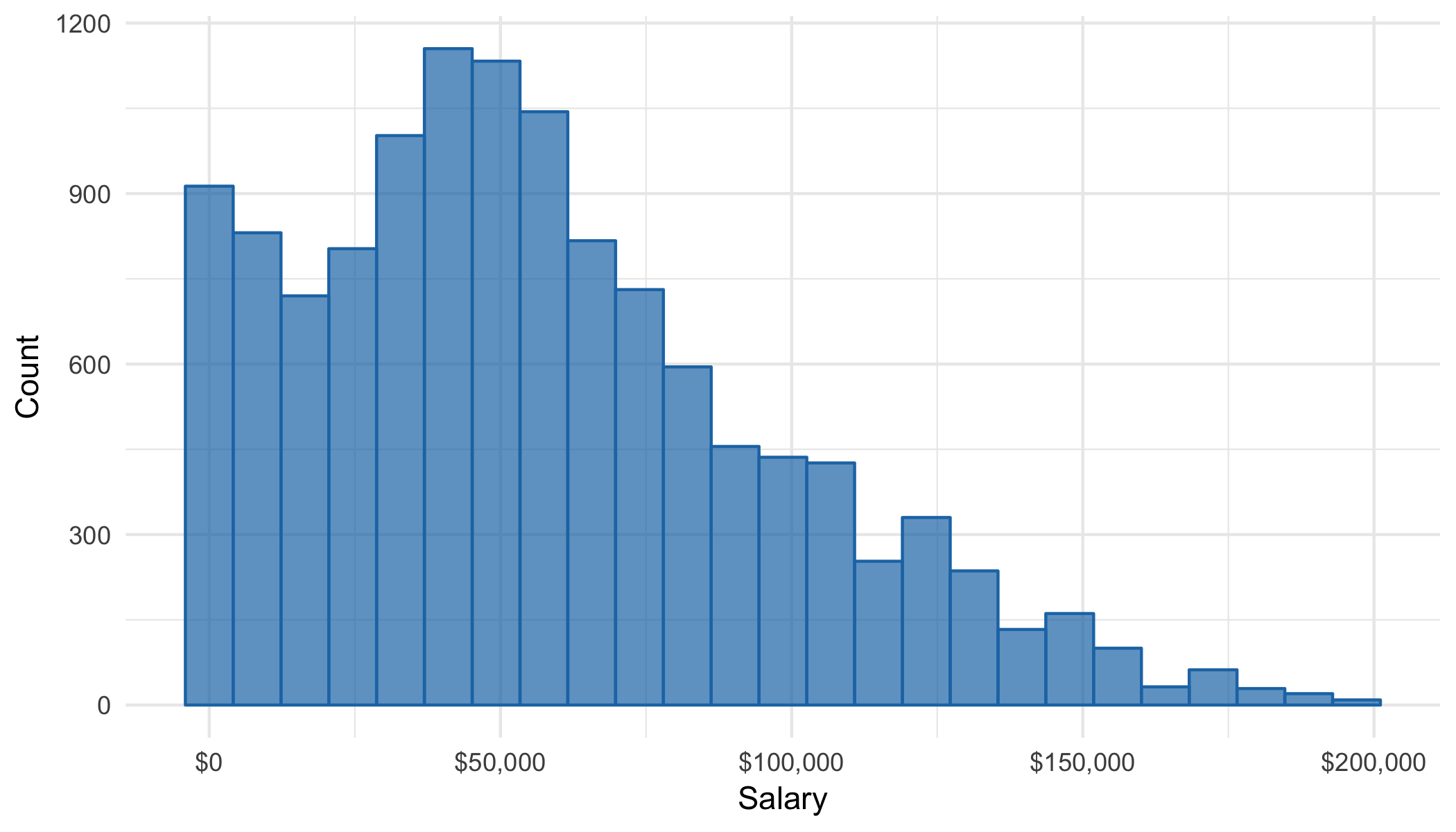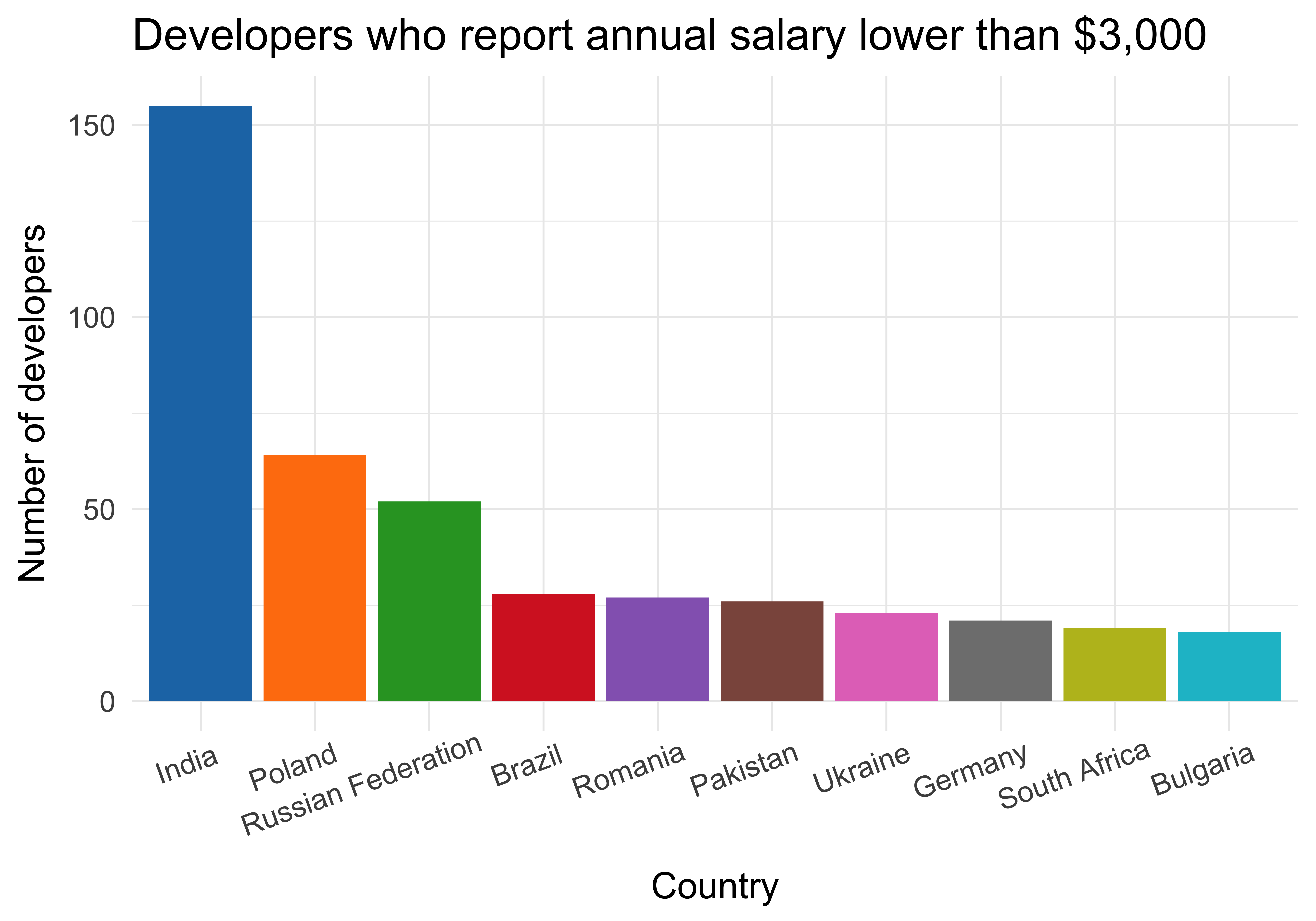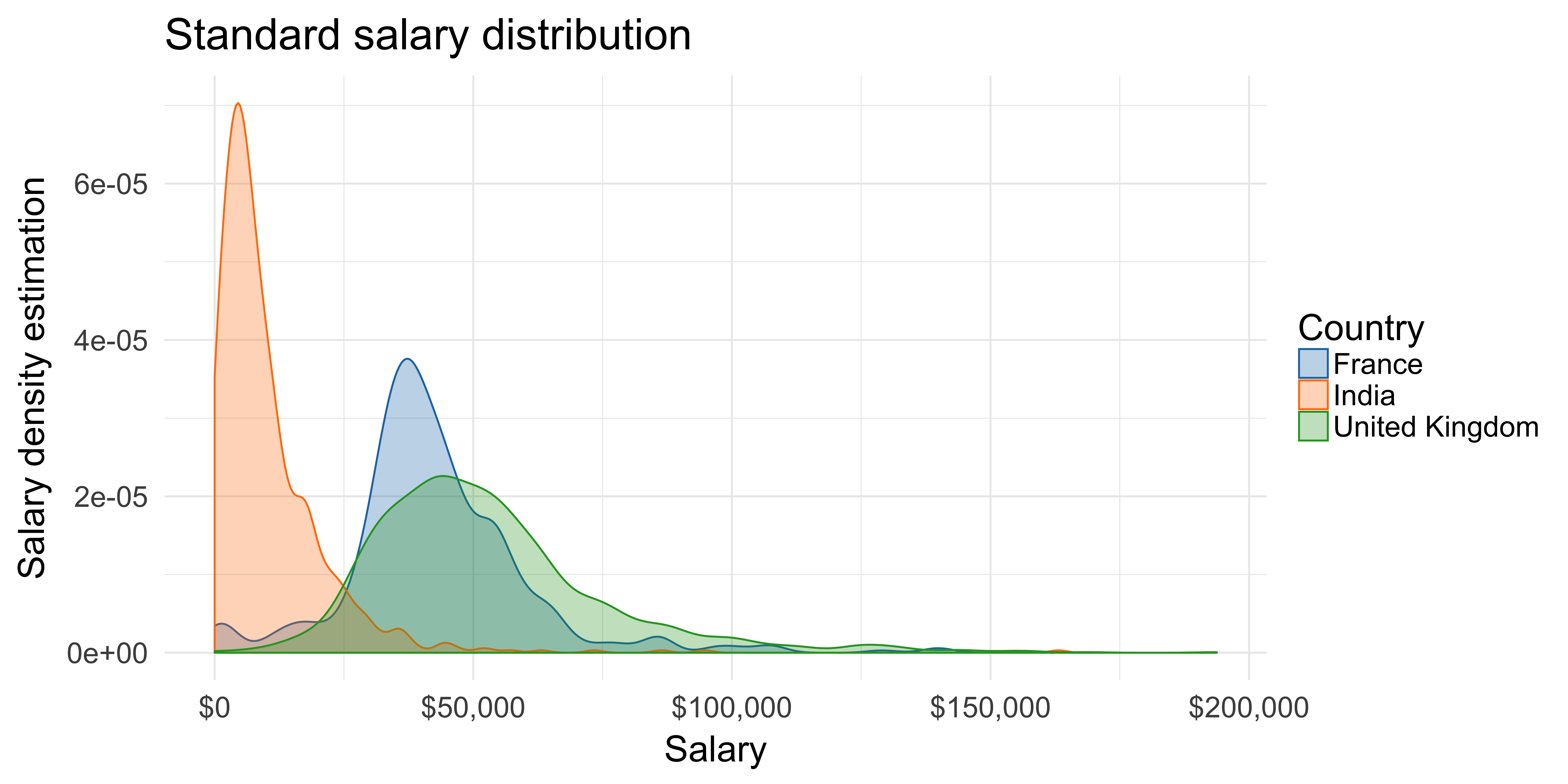# What's wrong?

What is your current annual salary, in [local currency]? Please enter a whole number in the box below, without any punctuation. If you prefer not to answer, please leave the box empty/blank.# Theory

## Mixture distributions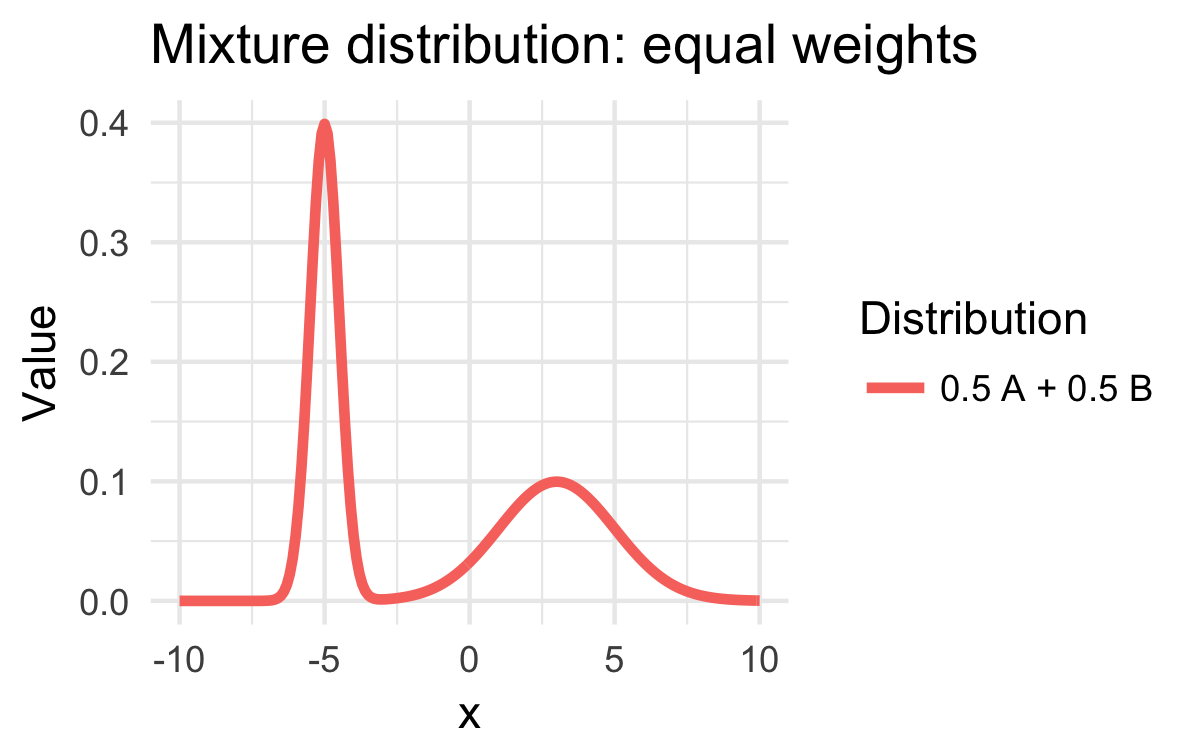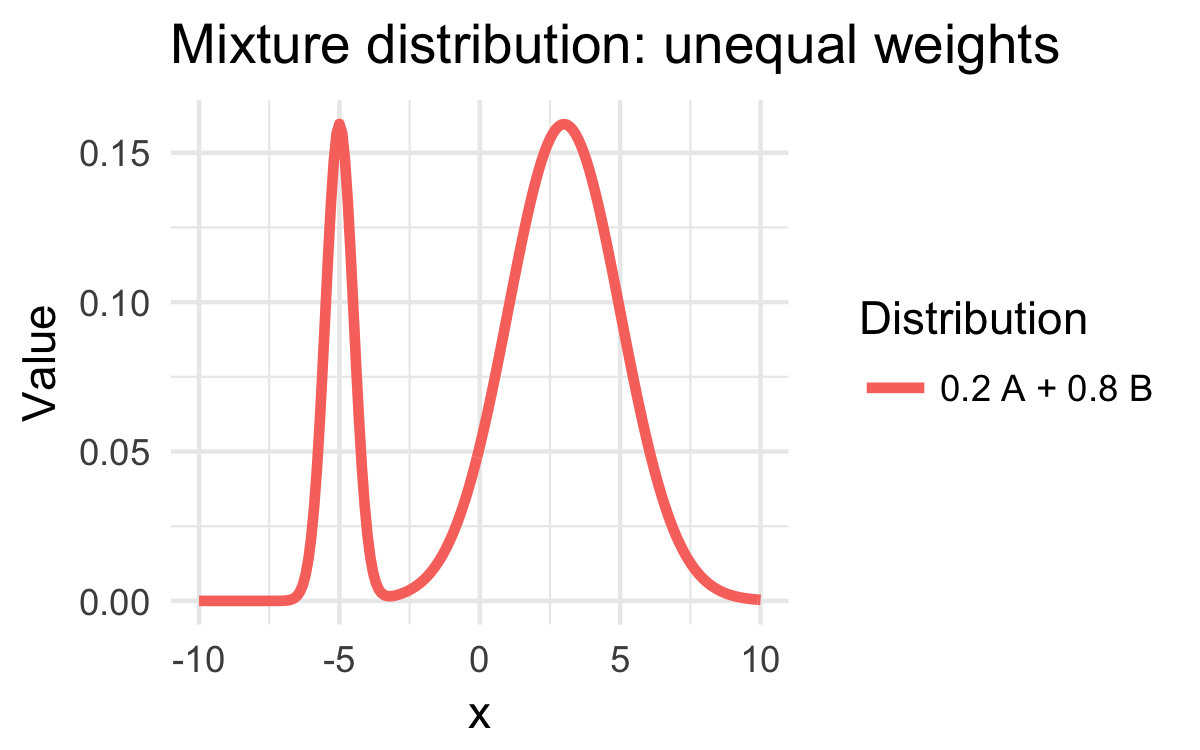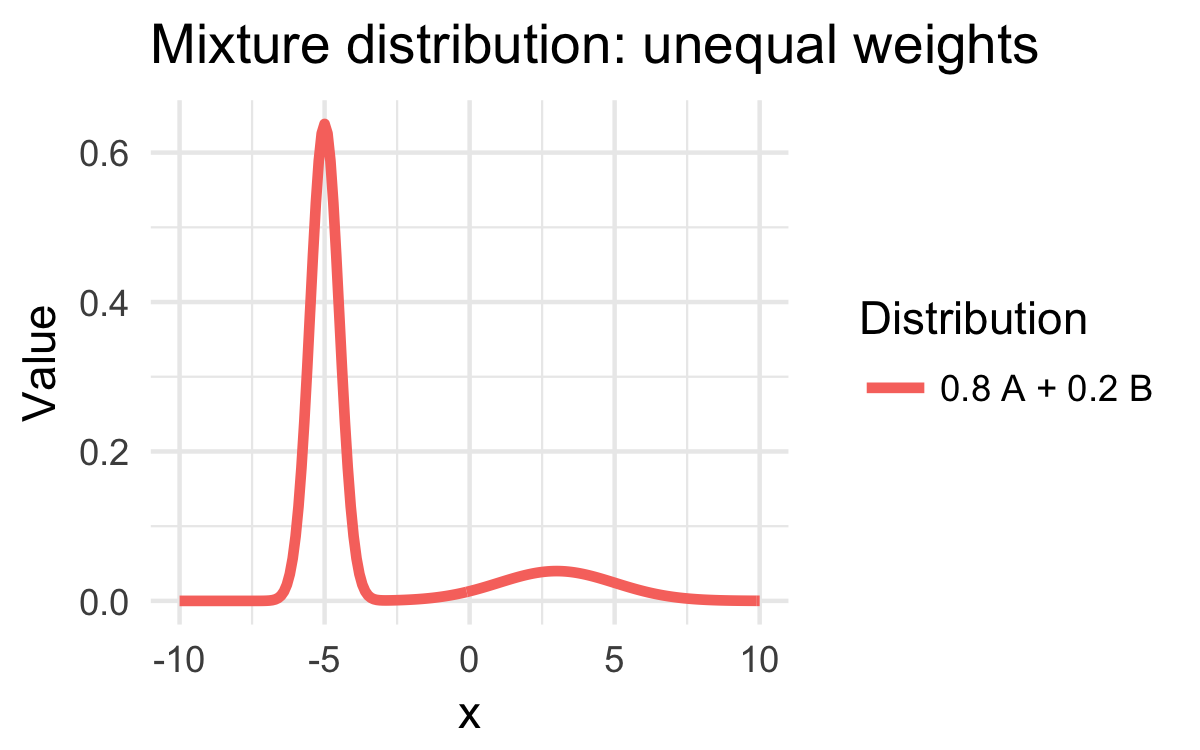# Probability distributions

## Mixture distribution: formally

$\text{Salary} = p \; \mathcal{N}\left(\mu, \sigma^2\right) + \left(1-p\right) \; \frac{1}{12} \; \mathcal{N}\left(\mu, \sigma^2\right)$

unknown: $$\mu$$, $$\sigma^2$$, $$p$$

# Monty Hall problem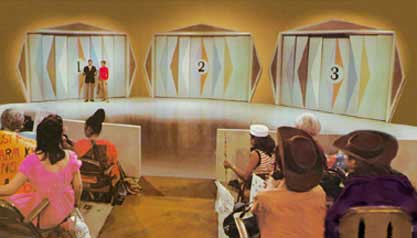## Mixture distribution: formally

$\text{Salary} = p \; \mathcal{N}\left(\mu, \sigma^2\right) + \left(1-p\right) \; \frac{1}{12} \; \mathcal{N}\left(\mu, \sigma^2\right)$

unknown: $$\mu$$, $$\sigma^2$$, $$p$$

## Mixture distribution: informally

$\text{Salary} = p(\text{correct}) \,\times\, \text{Annual salary} + \\ \;\;\;\;\;\;\;\;\; + \, p\left(\text{mistake}\right) \; \frac{1}{12} \,\times\, \text{Annual salary}$

unknown: $$p(\text{correct})$$, Annual salary

# Inference

## for mixture distributions

Easy if we knew the values of the unknown parameters

## Mixture distributions

### Probabilistic programming

 1: 2: 3: 4: 5: 6: 7: 8:  let salary = Gaussian(mean,variance) let mistake = Bernoulli(probability) let observed = if mistake then 1/12 * salary else salary 

# Modelling

## mixture distributions

But how do we get the parameters?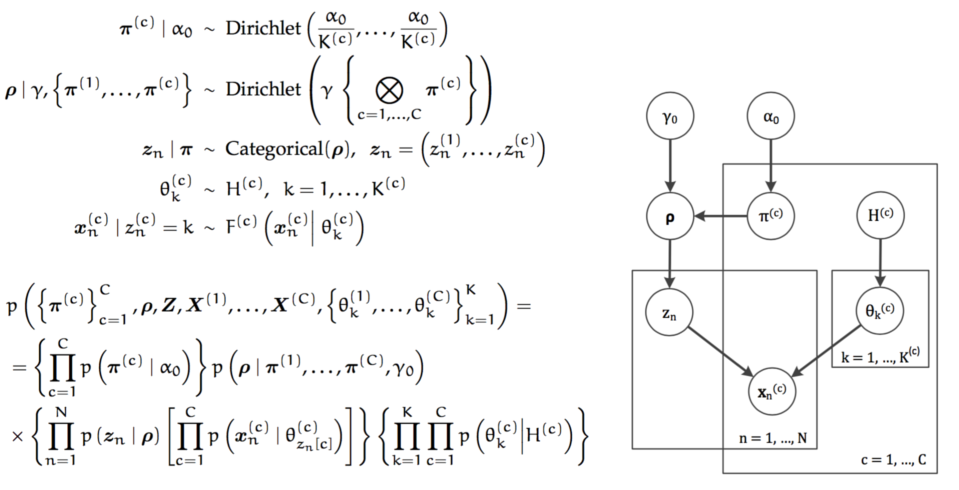## The world's slowest probability inference engine# complete enumeration### Try different parameter values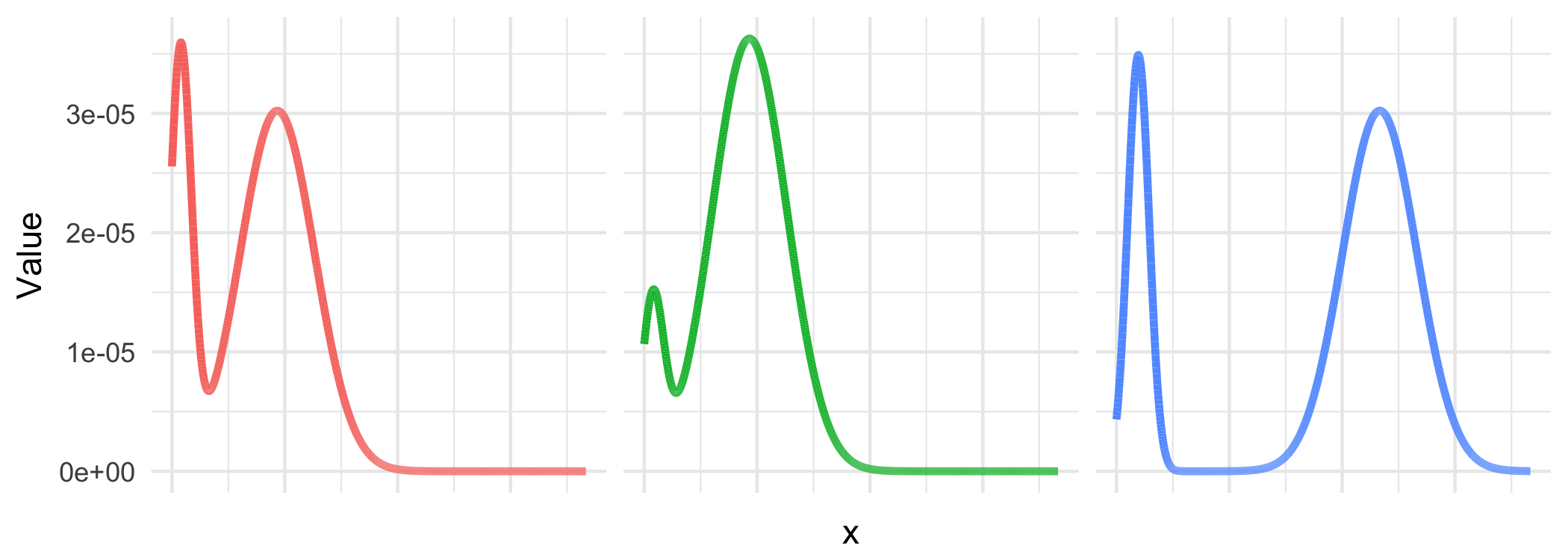### Discretize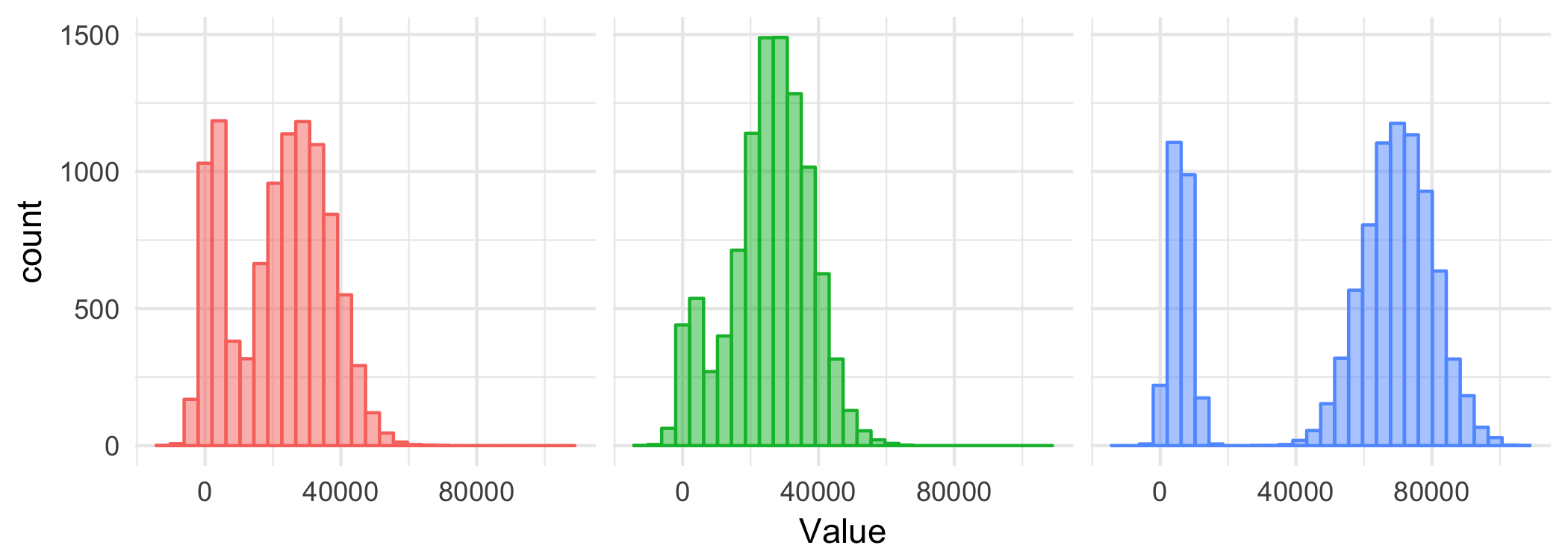### Compare two discrete distributions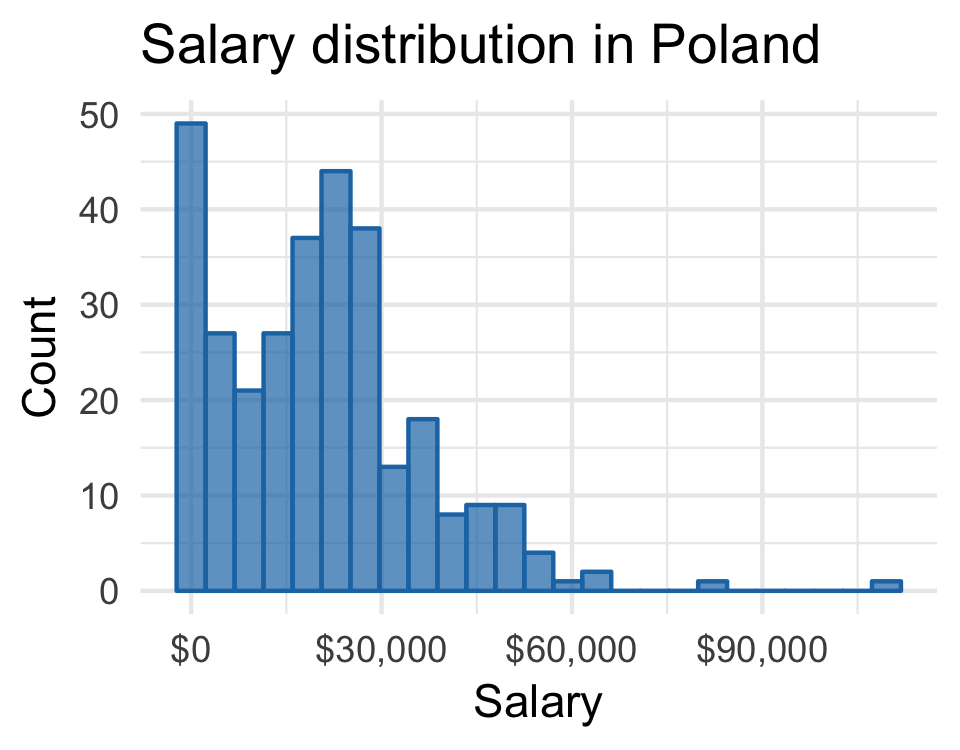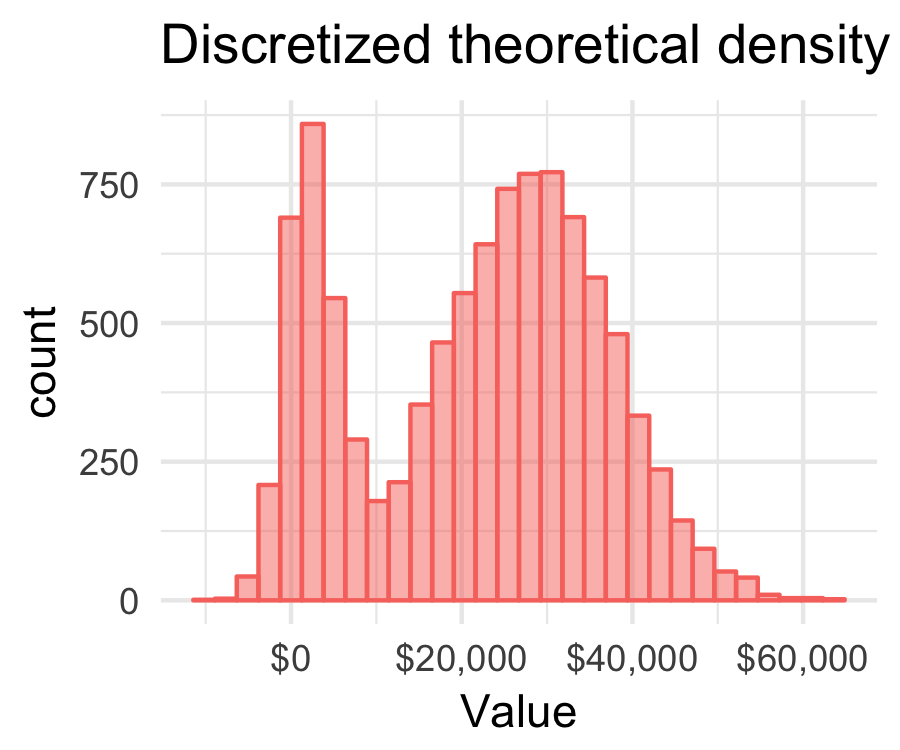## Demo

### The world's slowest probabilistic language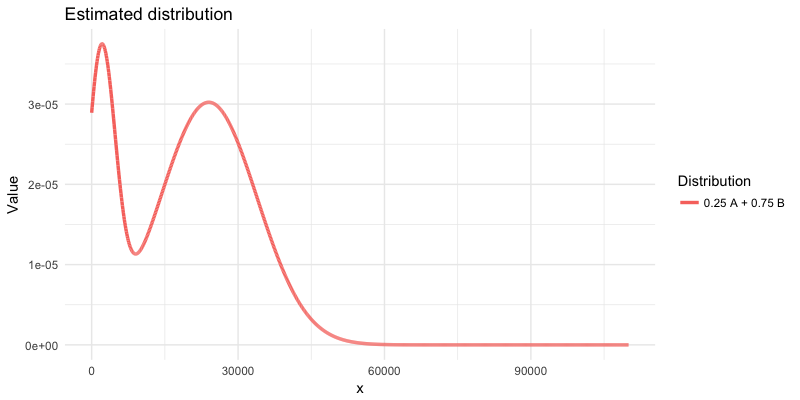# Probabilistic programming

## in the real world

  1: 2: 3: 4: 5: 6: 7: 8: 9: 10: 11: 12: 13: 14: 15: 16: 17: 18: 19: 20:   data { int N; vector[N] y; } parameters { vector mu; real sigma; real theta; } model { sigma ~ normal(0, 2); mu ~ normal(0, 2); theta ~ uniform(0.0,1.0); for (n in 1:N) target += log_mix(theta, normal_lpdf(y[n] | mu, sigma), normal_lpdf(y[n] | mu, sigma)); }